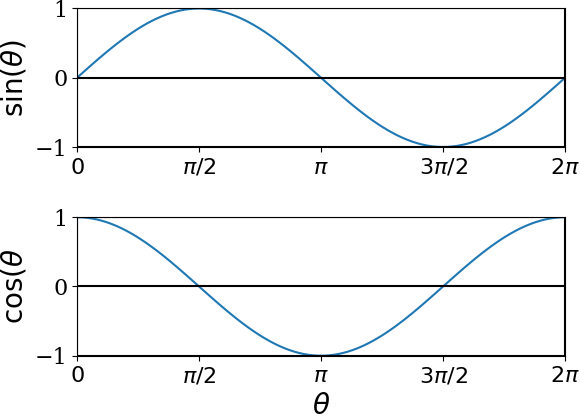## Section13.1Sine and Cosine Functions

You have seen sines and cosines in the context of triangles. There, we define them using the sides of a right-angled triangle.

\begin{align*} \amp \sin\,\theta = \dfrac{p}{h}, \\ \amp\cos\,\theta = \dfrac{b}{h}. \end{align*}In a right-angled triangle, the angle can go up to $90^{\circ}\text{.}$ More generally, the angle can be extended to the full $360^{\circ}$ in the plane, which we have used to indicate direction in a plane.

When we think sines and cosines as functions of $\theta\text{,}$ we usually view $\theta$ in radian and not in degrees with $360^{\circ}$ equal to $2\pi$ radian. Furthermore, we extend the range of values for $\theta$ to any real value, $-\infty \lt \theta \lt \infty \text{,}$ and require that sines and cosines be periodic in $\theta$ with period $2 \pi\text{.}$

\begin{align*} \amp \sin\,(\theta + 2\pi m) = \sin\,\theta, \ \ \ (m\text{ any integer}) \\ \amp\cos\,(\theta + 2\pi n) = \cos\,\theta, \ \ \ (n\text{ any integer}) \end{align*}

This periodicity of sine and cosine functions makes them valuable for representing vibrations and waves, such as sound and light.Figure 13.1.1. One period of sine and cosine functions in the range $[0,2\pi]\text{.}$ You can extend these plots to any real value of $\theta$ by adding or subtracting interger multiples of $2 \pi\text{.}$ Say you want $\sin(20)\text{,}$ you first divide $20$ by $2\pi\text{,}$ and throw away the integer part. That gives you $0.183098861837907\text{,}$ which you can multiply by $2\pi$ to get $1.15 \text{,}$ whose sine will be 0.913 in this plot.

The uniform circular motion in the $xy$ plane has two harmonic motions inside it - one along the $x$ axis and one along the $y$ axis. Here is website that you can play aroud interactively Sine and Cosine in Uniform Motion in a Unit Circle or go to "https://www.desmos.com/calculator/cpb0oammx7".